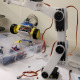# How to handle multi communications (I2C SPI UART) in single program of arduino

2 posts / 0 newOffline

Joined: May 22, 2018

Replies: 0
How to handle multi communications (I2C SPI UART) in single program of arduino
May 22, 2018 - 5:34pm

I am doing one project using ARDUINO UNO, which is ADC(ADS1115, 3 analog inputs) data and GPS(NEO-6M-0-001) data to store in microSD card.

Here the the GPS contains UART(Tx, Rx) communication, SD card contains SPI communication and ADS1115 contains I2C communication.

Here when i'm connected GPS and Sd card, The GPS data is stored in SD card and Like this when i'm connecting ADS1115 and SD card the data is stored in SD card

But the problem is while i'm connecting all (GPS, ADS1115, SD card) at a time it is not storing data in sd card. (Some one told me that is using of different communications in single program)

how to solve this issue ?

i tagged my code also please find it.
<CODE>

#include <TinyGPS++.h>                                  // Tiny GPS Plus Library
#include <SoftwareSerial.h>                             // Software Serial Library so we can use other Pins for communication with the GPS module
TinyGPSPlus gps;                                        // Create an Instance of the TinyGPS++ object called gps
static const uint32_t GPSBaud = 9600;
#include <SPI.h>
#include <SD.h>
#include <Wire.h>

SoftwareSerial ss(6, 5);                                // The serial connection to the GPS device

void setup()
{
Serial.begin(9600);                                 // Update display
while (!Serial) {
; // wait for serial port to connect. Needed for native USB port only
}
//delay(500);                                          // Pause in milli seconds
ss.begin(GPSBaud);                                    // Set Software Serial Comm Speed to 9600
// set up the LCD's number of columns and rows:
// lcd.begin(16, 2);
// lcd.print("Flux gate magnetometer");
if (!SD.begin(4)) {
//   lcd.print("sd card initialization failed");
Serial.println("Card failed, or not present");
return;
}

}
void loop()
{
/////////////////
float flat = gps.location.lat();
float  flon = gps.location.lng();
/////////////////
float xdir, ydir, zdir, Tfield;
/////////////////
xdir = adc0 * (0.1875 / 1000) / 0.000025;
ydir = adc1 * (0.1875 / 1000) / 0.000025;
zdir = adc2 * (0.1875 / 1000) / 0.000025;
Tfield = sqrt(sq(adc0 * (0.1875 / 1000)) + sq(adc1 * (0.1875 / 1000)) + sq(adc2 * (0.1875 / 1000))) / 0.000025;
//////////////////
File myFile;
/////////////////////
while (ss.available() > 0) {
}
if (gps.location.isUpdated()) {
myFile = SD.open("test2.txt", FILE_WRITE);
if (myFile) {
myFile.print(F("  Date/Time: "));
// lcd.print(F("  Date/Time: "));
myFile.print(gps.date.month());
myFile.print(F("/"));
myFile.print(gps.date.day());
myFile.print(F("/"));
myFile.print(gps.date.year());
myFile.print(F("/"));
if (gps.time.isValid()) {
if (gps.time.hour() < 10) Serial.print(F("0"));
myFile.print(gps.time.hour());
myFile.print(F(":"));
if (gps.time.minute() < 10) Serial.print(F("0"));
myFile.print(gps.time.minute());
myFile.print(F(":"));
if (gps.time.second() < 10) Serial.print(F("0"));
myFile.print(gps.time.second());
myFile.print(F(":"));
if (gps.time.centisecond() < 10) Serial.print(F("0"));
myFile.println(gps.time.centisecond());
myFile.print(F(" "));
myFile.print("Latitude  : ");
myFile.println(flat, 6);
myFile.print(F(" "));
myFile.print("Longitude : ");
myFile.println(flon, 6);
myFile.print(F(" "));
myFile.print("fieldX in nT: ");  myFile.println(xdir);
myFile.print(F(" "));
myFile.print("fieldY in nT: ");  myFile.println(ydir);
myFile.print(F(" "));
myFile.print("fieldZ in nT: ");  myFile.println(zdir);
myFile.print(F(" "));
myFile.print("Total field in nT: ");  myFile.println(Tfield);

}
}
myFile.close();
}

myFile = SD.open("test2.txt");
if (myFile) {
// read from the file until there's nothing else in it:
if (myFile.available()) {
}
// close the file:
myFile.close();
} else {
// if the file didn't open, print an error:
Serial.println("error opening test1.txt");
}
}Offline

Joined: Aug 16, 2016

Replies: 996

Hi,

It is defiantly possible to use more than one communication protocol on a single Microcontroler. It is not possible to go through your code and find out what the problem is unless you brief about the problem you are having. But to give you an idea...

Multiple UART on Arduino:

Multiple serial devices like GPS, GSM or Bluetooth module can be connected to Arduino, by using the Software serial library. This library, allows us to convert any normal GPIO pins on the Arduino into a Tx and Rx pin.

Multiple I2C devices on Arduino:

To connect multiple I2C, we have to address each slave device on the I2C bus. I assume you are using the Arduino as master and the other devices as slave. In that case each slave device will have an unique I2C address using which we can communicate with the respective device. The SCL and SDA lines will be connected to all the slave devices

Multiple SPI devices on Arduino:

Multiple SPI devices can be connected to Arduino by using the CS (SS) pins.  The MISO, MOSI and clock pin will be connected to all the slave devices from the Arduino. Then the we use another pin called CS (Chip Select) which is connected to different GPIO pins of the Arduino. This pin has to be held low if we want to communicate with that particular pin.

Hope it helps!